# Some Special Parallelograms Class 8 Notes | EduRev

## Class 8 : Some Special Parallelograms Class 8 Notes | EduRev

The document Some Special Parallelograms Class 8 Notes | EduRev is a part of the Class 8 Course Class 8 Mathematics by VP Classes.
All you need of Class 8 at this link: Class 8

SOME SPECIAL PARALLELOGRAMS
1. Rectangle
A rectangle is a parallelogram in which each angle is a right angle.
In rectangle,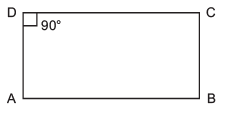(i) The opposite sides are equal and parallel,
(ii) Opposite angles are equal,
(iii) Diagonals ae equal,
(iv) Diagonals bisect each other.
Note: A rectangle is an equiangular figure but not an equilateral one.

2. Rhombus
Arhombus is a parallelogram in which all four sides are equal.
In a rhombus,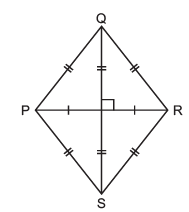(i) Opposite sides are parallel,
(ii) All sides are equal,
(iii) Opposite angles are equal,
(iv) Diagonals bisect each other at right angles.

3. Square
A parallelogram having all of its sides equal and measure of each angle being 90°, is called a square.
In a square,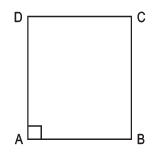(i) All four sides are equal,
(ii) Opposite sides are parallel,
(iii) Each angle being equal to 90°,
(iv) The diagonals are equal,
(v) The diagonals bisect each other at right angles.
Note: A square is an equilateral and equiangular quadrilateral. Therefore it is called a regular polygon.

SUM OF THE MEASURES OF THE EXTERIOR ANGLES OF A POLYGON
Whatever be the number of sides of a polygon, the sum of the measures of its exterior angles is always 360°.
For example:
(a)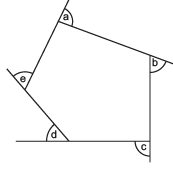a + ∠b + ∠c + ∠d + ∠e = 360°

(b)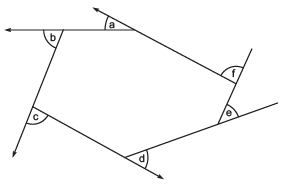a + b + c + d + e + f = 360°

Example 1. Find the measure of x in the figure.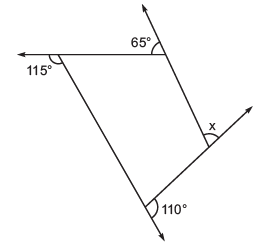Solution:
∵ Sum of all the exterior angles of a quadrilateral = 360°
∴ x + 65° + 115° + 110° = 360°
or
x + 290° = 360°
or
x = 360° – 290° = 70°

Offer running on EduRev: Apply code STAYHOME200 to get INR 200 off on our premium plan EduRev Infinity!

93 docs|16 tests

,

,

,

,

,

,

,

,

,

,

,

,

,

,

,

,

,

,

,

,

,

;